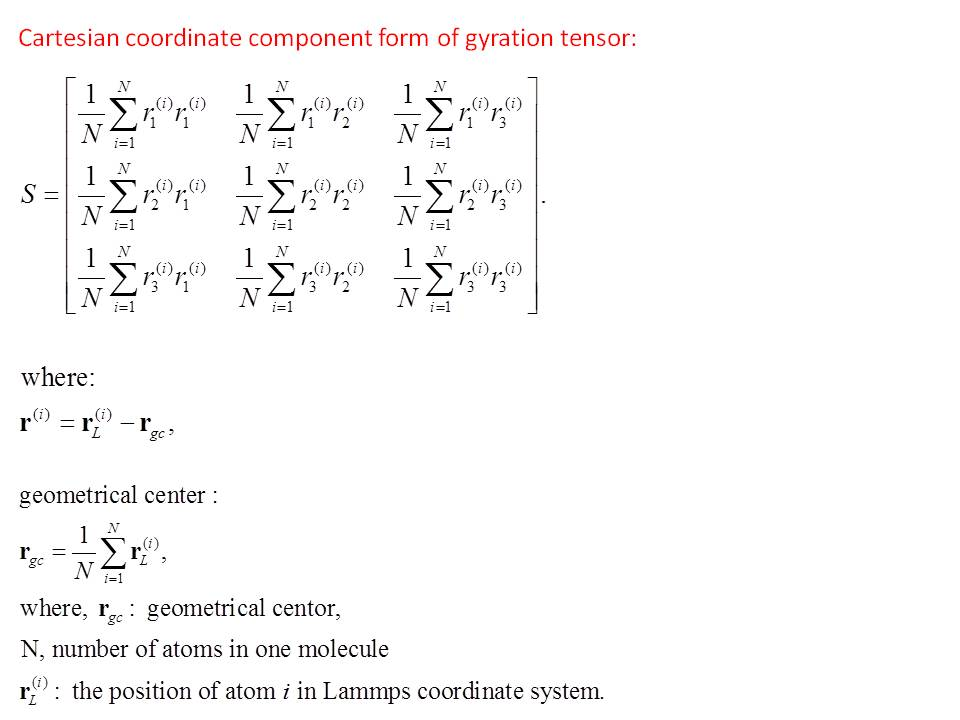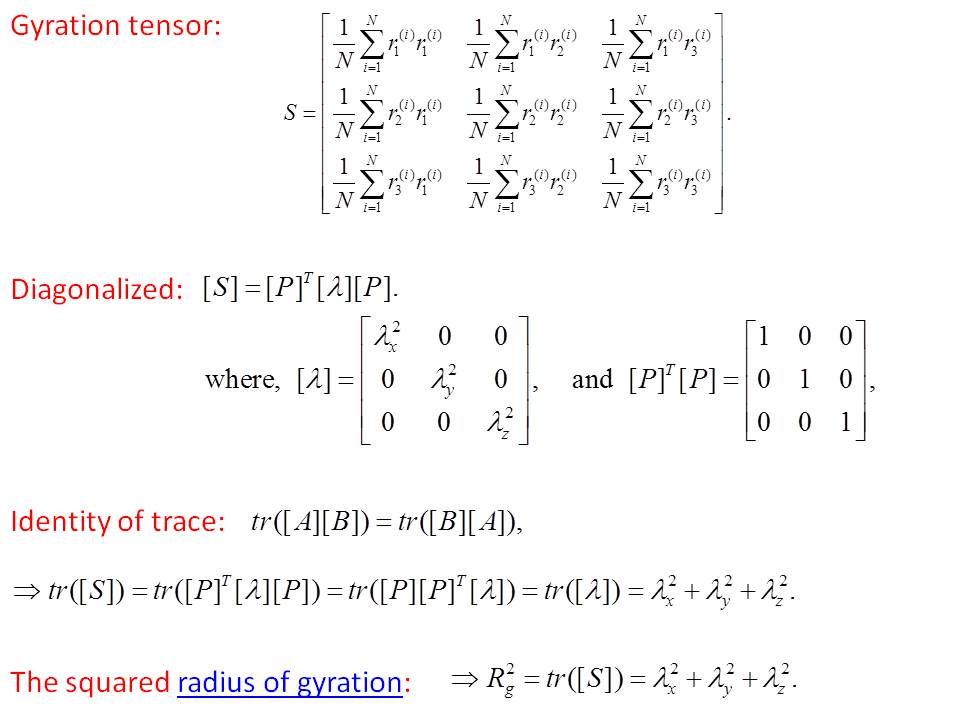# Output Gyration tensor

Dear Everyone,

I want to study the conformation property changes of polymer chains during deformations.
Is there any command or utility package in Lammps that can output Gyration tensor of the molecules directly?

Lili Zhang

Dear Everyone,

I want to study the conformation property changes of polymer chains during
deformations.
Is there any command or utility package in Lammps that can output Gyration
tensor of the molecules directly?

not at the time or unless you write it. all there is is:
http://lammps.sandia.gov/doc/compute_gyration.html

axel.

There is also a compute gyration/molecule which will calcalate
Rg on a per-molecule basis.

Steve

Dear Steve and Axel,

It seems that we have to calculate the tensor form of the gyration for each molecule from the Lammps output by ourselves. Since I want to study how the polymer chains change 3 dimensionally when I apply large deformations.

But I’m worried that because of the periodic boundaries, some molecules (or atoms) might cross the boundaries and thus their coordinates won’t be in the same frame as the center of mass, so that I will loose some atoms’ coordinates that belong to the molecule under study.

Any suggestions on how to solve this kind of problem?

Thank you for your time and help,

Lili

Check out NEMD part in the manual:

http://lammps.sandia.gov/doc/Section_howto.html#howto_13

Requires fair amount of understanding, Allen and Tidesley would be the
best bet !!

Dear Steve and Axel,

It seems that we have to calculate the tensor form of the gyration for each
molecule from the Lammps output by ourselves. Since I want to study how the
polymer chains change 3 dimensionally when I apply large deformations.

But I'm worried that because of the periodic boundaries, some molecules (or
atoms) might cross the boundaries and thus their coordinates won't be in the
same frame as the center of mass, so that I will loose some atoms'
coordinates that belong to the molecule under study.

Any suggestions on how to solve this kind of problem?

just write an unwrapped trajectory

dump_modify <dumpid> unwrap yes

if in the original system the molecules are properly
placed and "whole", then they should remain like
this over the course of the trajectory.

axel.

Is the gyration tensor simply the formula on the
compute gyration doc page with (r-rcm)^2
replaced by (x-xcm)(y-ycm) for the xy component,
etc?

If so, it would be very simple to extend the
2 gyration computes to calculate the tensor
as well as Rg. They account for PBC, etc.

Steve

Dear Steve,

Yes, the equation for gyration tensor is as what you mentioned.

“The extension of the 2 gyration computes” you meant is that we can modify the corresponding parts in the Lammps source code, so that the output of our new compute will directly give the gyration tensor components??
If so, I think it will be better than my original plan which is to work on the coordinates output of the atoms in individual molecule, then write some code to calculate the tensor, that is why I thought maybe I will loose some atoms’ coordinates due to period boundary conditions.

Lili

yes, I mean the 2 computes could be extended with a bit of code to also
calculate the 6 tensor values (I assume it is symmetric).

Steve

Just added this as a 13Jun12 patch.
Try it out and see if it computes what you want.

Steve

Dear Steve and Lammps Users,

Here is my feedback about using the new compute command you added last week:

I am attempting to output the tensor components of the radius of gyration using the ‘compute gyration/molecule tensor’ command. To do so, I’m taking an average of all molecules, using this script:

compute RG all gyration/molecule tensor

variable RG1 equal ave(c_RG)
variable RG2 equal ave(c_RG)
variable RG3 equal ave(c_RG)
variable RG4 equal ave(c_RG)
variable RG5 equal ave(c_RG)
variable RG6 equal ave(c_RG)

I get values for the first three (xx, yy, zz), but the last three components (xy, xz, yz) are outputted as “-nan”. Our box is orthogonal, could that have anything to do with the lack of outputs?"

Thank you,
Lili

I think the issue, which I didn't think about, is
that the cross terms (4,5,6) can be negative
and the code is taking the sqrt() of them.
What is the right thing to do with these values?
Maybe the sqrt(abs(value)) and tack the +/- sign
back on?

Steve

I think the issue, which I didn't think about, is
that the cross terms (4,5,6) can be negative
and the code is taking the sqrt() of them.
What is the right thing to do with these values?
Maybe the sqrt(abs(value)) and tack the +/- sign
back on?

where does the square root enter?

http://en.wikipedia.org/wiki/Gyration_tensor

axel.

Hello Steve,

Please see the attached file for the gyration tensor form and the geometrical center which is used to calculate it. The forms I found are from one paper studying the shape of polymer chains.

Liliok - I removed the sqrt() - will post a patch this AM.

What confused me is that the scalar Rg does take a sqrt()
of the final result.

One potential issue, from the Wiki page that Axel
posted. The scalar Rg there is the trace of the diagonalized
gyration tensor. Whereas what LAMMPS does is simply
the trace of the undiagonalized tensor, I believe. Namely
sqrt(sum(dx^2 + dy^2 + dz^2)). This seems like it would in general be
a different answer, unless the tensor is trace invariant
to diagonalization? Comment ??

Steve

Dear Steve,

Please see the attached file for the relation. The answer will be the same.

Thank you much for your help,
Liligood to know - makes sense now

Steve